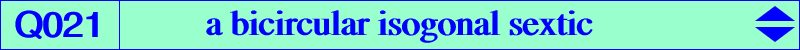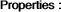too complicated to be written here. Click on the link to download a text file.X(1), X(3), X(4), X(1113), X(1114), X(2574), X(2575), X(5000), X(5001) excenters anti-points, see Table 77 Ha, Hb, Hc : vertices of the orthic triangle Ka, Kb, Kc : feet of the Lemoine axis (centers of the apollonian circles) foci of the K-ellipse (inellipse with center K when the triangle ABC is acute angle) E1, E2 see belowQ021 is a bicircular isogonal sextic. It is the locus of P such that the orthotransversals of P and its isogonal conjugate P* are parallel. See also Q022. Another description is the following : P, H and the orthocenter of the circumcevian triangle of P are collinear if and only if P lies on Q021 (together with the line at infinity). Compare Q021 and Q019, Q024. See also Panchapakesan isogonal sextics at Q170.A, B, C are double points. The tangents at A are the line AO and the parallel at A to BC. The tangents at Ha, Hb, Hc concur at X(5) not on the curve. The tangents at the four in/excenters concur at X(1498) not on the curve. Q021 meets the Euler line at O, H, X(1113), X(1114) (these two latter points on the circumcircle) and two (not always real) other points E1, E2 on the circle centered at X(468) orthogonal to the circumcircle. These are X(5001), X(5000) respectively. The tangents at O and H pass through X(68). Q021 has two real asymptotes parallel to those of the Jerabek hyperbola. They meet at the point X(11064) = SA [ (b^2-c^2)^2+a^2(b^2+c^2-2a^2)] : : , on the line GK.